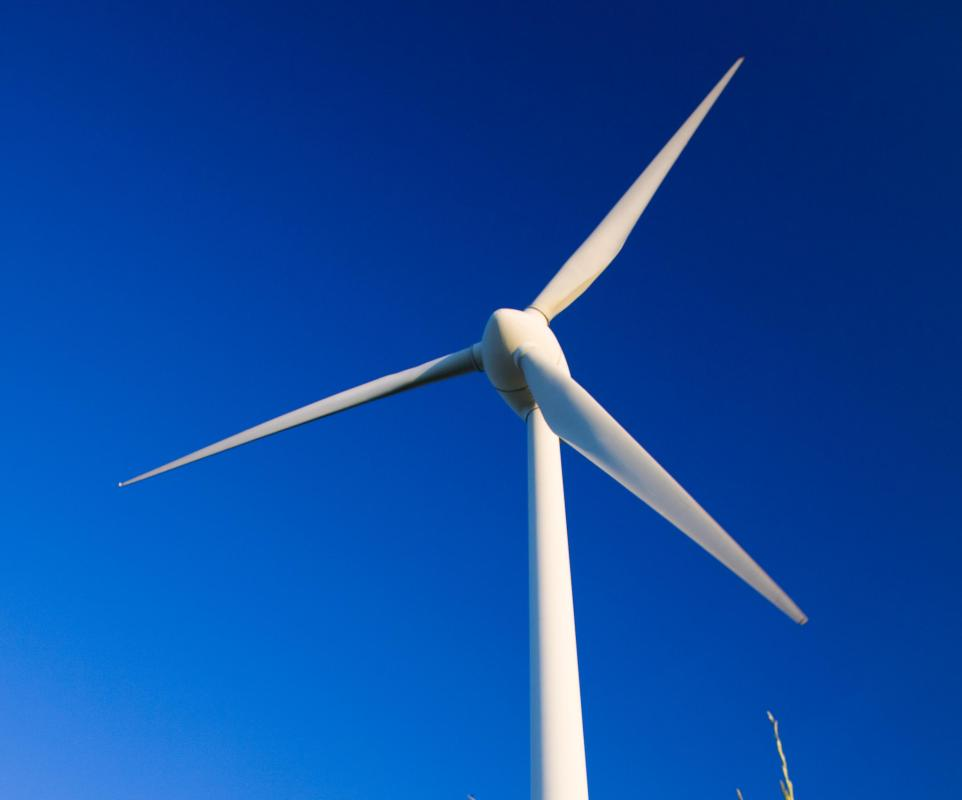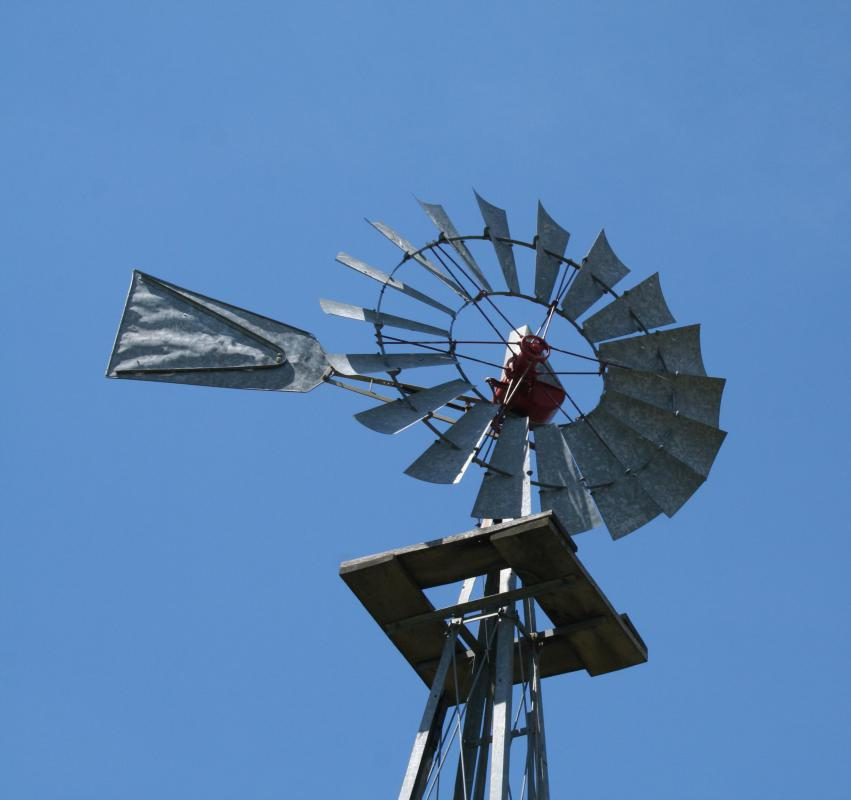What is Wind Potential Energy?

Larry Ray Palmer
Larry Ray PalmerWind potential energy is converted to kinetic energy -- and electricity -- with a turbine.

Potential energy was first defined in the 19th century by Scottish physicist William Rankine. He explained it as energy stored within a system that could be converted to work. When referring to wind potential energy, this can be understood as the amount of usable energy that may be feasibly produced by wind currents. Such wind energy is converted to kinetic energy through such means such as wind turbines, wind mills, wind pumps, and the sails of ships. Humankind has a long history of using wind's potential energy for its own purposes: to power wind mills, to grind grain, to push sailing ships across the sea and, more recently, to produce electricity.Wind's energy has been used for centuries to pump water and grind grain.

Wind potential energy is measured in joules. A joule is defined as the work needed to produce one watt of power for a one second period. The basic theory behind wind potential energy states that wind at a certain speed and strength can create enough joules of energy to perform a certain task. For example, if a steady breeze turns a wind turbine's blades 10 revolutions per second, and each revolution produced 1.5 joules, then the resulting wind potential energy would be 15 joules. This means that it could reasonably produce 15 watts of energy every second.

Using this same example, if the wind continued at the same steady breeze for one hour, it would produce 54 kilowatts of electricity. The potential energy of the breeze would be 54,000 joules per hour. Such a figure could power a 100 watt light bulb continuously for 540 hours before being depleted.

The formula for figuring wind potential energy is also used to assess the economic feasibility of wind turbine fields and other wind energy collection methods. In areas where the wind is steady, it can be an economically sound decision to try to harness its power. In areas where the air movement is less than steady, however, the expense involved in setting up and maintaining wind turbines and other devices to harness energy may outweigh any benefits that could be gained.

To determine economic feasibility, the formula used to obtain the wind potential energy from the previous example is used and then multiplied by the number of proposed wind turbines. Using the previous example, if a single wind turbine produces 54,000 joules of electrical energy per hour, ten wind turbines can reasonably be expected to have a wind potential energy of 540,000 joules per hour. By this estimation, the wind potential energy of such a wind turbine field will have the potential to produce 540 kilowatt-hours of electricity per hour for as long as the wind continues.

You might also Like